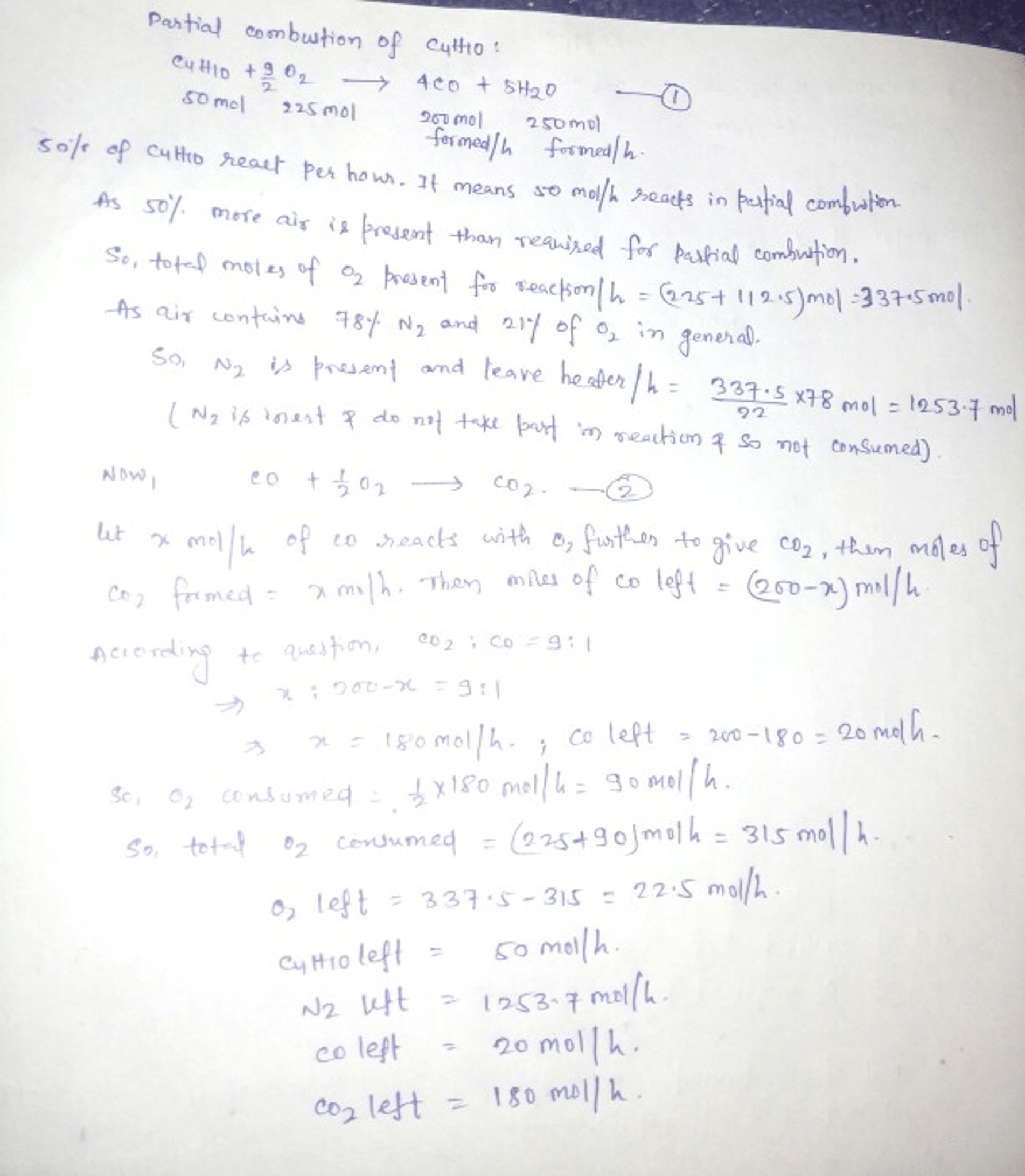# Butane (C4H10) is fed to a fired heater at a molar flow rate of 100 mol/h and 50% excess air. Fifty percent of the butane is consumed, and the product gas contains 9 mol CO2 per mol CO. Calculate the molar flow rate of water in the stack gas.

Question-AnswerCategory: Material And Energy BalanceButane (C4H10) is fed to a fired heater at a molar flow rate of 100 mol/h and 50% excess air. Fifty percent of the butane is consumed, and the product gas contains 9 mol CO2 per mol CO. Calculate the molar flow rate of water in the stack gas.

Butane (C4H10) is fed to a fired heater at a molar flow rate of 100 mol/h and 50% excess air.
Fifty percent of the butane is consumed, and the product gas contains 9 mol CO2 per mol
CO. Calculate the molar flow rate of water in the stack gas.